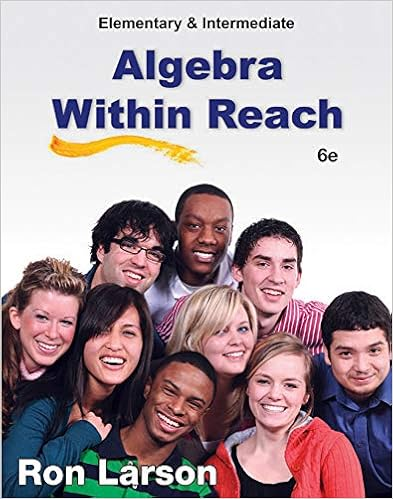# Consider the event that the randomly selected student

• Notes
• 19
• 100% (1) 1 out of 1 people found this document helpful

This preview shows page 12 - 16 out of 19 pages.

##### We have textbook solutions for you!
The document you are viewing contains questions related to this textbook.The document you are viewing contains questions related to this textbook.
Chapter 3 / Exercise 51
Elementary and Intermediate Algebra: Algebra Within Reach
LarsonExpert Verified
Consider the event that the randomly selected student is a graduate student who uses a Mac. Write this event in symbolic form using the two events of interest that chose in b.d.Find the probability of the event described c. Explain your method.DAY 4
##### We have textbook solutions for you!
The document you are viewing contains questions related to this textbook.The document you are viewing contains questions related to this textbook.
Chapter 3 / Exercise 51
Elementary and Intermediate Algebra: Algebra Within Reach
LarsonExpert Verified
CHAPTER 5PART 3– Conditional Probability and IndependenceOBJECTIVE(S):Students will learn how to use a tree diagram to describe chance behavior.Students will learn how to use the general multiplication rule to solve probability questions.Students will learn how to compute conditional probabilities.Students will learn how to determine whether two events are independent.Students will learn how to find the probability that an event occurs using a two-way table.Students will learn how to use the multiplication rule for independent events to compute probabilities.Conditional Probability -Independent Events -General Multiplication Rule -16. In 1912 the luxury liner Titanic, on its first voyage across the Atlantic, struck an iceberg and sank. Some passengers got off the ship in lifeboats, but many died. The two-way table gives information about adult passengers who lived and who died, by class of travel. Suppose we choose an adult passenger at random.Survival StatusClass of TravelSurvivedDiedFirstClass197122SecondClass94167ThirdClass151476a.Given that the person selected was in first class, what’s the probability that he or she survived?
CHAPTER 5b.If the person selected survived, what’s the probability that he or she was a third-class passenger?c.Find(29| secP survivedond class.d.Find(29P survived.e.Determine whether the events “survived” and “second class” are independent. Explain your reasoning.17. The two-way table describes the 595 students who responded to a school survey about eating breakfast. Suppose we select a student at random. Consider events B: eats breakfast regularly, and M: is male.MaleFemaleTotalEatsbreakfastregularly190110300Doesn’teatbreakfastregularly130165295Total320275595a.Find(29|P B M. Explain what this value means.b.Find(29|P MB. Explain what this value means.c.Are the events B and M independent? Justify your answer.
CHAPTER 518. Here is the distribution of the adjusted gross income (in thousands of dollars) reported on individual federal income tax returns in a recent year:Income<2525-4950-99100-499500Probability0.4310.2480.2150.1000.006a.What is the probability that a randomly chosen return shows an adjusted gross income of \$50,000 or more?b.Given that a return shows an income of at least \$50,000, what is the conditional probability that the income is at least \$100,000?19. A shipment contains 10,000 switches. Of these, 1,000 are bad. An inspector draws 2 switches at random, one after the other. a.Draw a tree diagram that shows the sample space of this chance process.b.Find the probability that both switches are defective.
•••